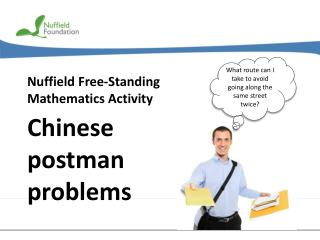DownloadDownload PresentationNuffield Free-Standing Mathematics Activity

# Nuffield Free-Standing Mathematics Activity

Download Presentation## Nuffield Free-Standing Mathematics Activity

- - - - - - - - - - - - - - - - - - - - - - - - - - - E N D - - - - - - - - - - - - - - - - - - - - - - - - - - -
##### Presentation Transcript

1. What route can I take to avoid going along the same street twice? Nuffield Free-Standing Mathematics Activity Chinese postman problems

2. College Open Day What route can I take to avoid going along the same street twice? Chris is delivering leaflets to houses near the college. The leaflets tell residents about the college open day and apologises for any inconvenience caused. Chris wants to deliver the leaflets as efficiently as possible. This is an example of a Chinese postman problem. In this activity you will learn how to solve such problems.

3. A College (C) B D E F G The map shows streets near the college. Think about Is it possible to deliver leaflets to the houses in these streets without travelling along the same street twice?

4. Think about Which of the graphs above can be traced without taking your pen from the paper? The college map is an example of a graph. Here are some others. A graph is made up of a collection of vertices called nodes, joined by edges called arcs. Atraversablegraph is one that can be drawn without taking a pen from the paper and without retracing the same edge

5. A Swiss mathematician, Leonhard Euler (1707 to 1783), published the first paper on graph theory in 1736. The paper was based on the Seven Bridges of Konigsberg problem. Residents of Konigsberg wanted to know if it was possible to find a route which passed across each of the bridges only once.

6. Euler found that the order of the vertices determines whether or not a graph is traversable. If it is possible to traverse a graph starting and finishing at the same point then the graph has an Eulerian trail. If it is possible to traverse a graph starting at one point and finishing at a different point then the graph has a semi-Eulerian trail.

7. order 1 order 2 order 3 order 4 order 6 order 5 Odd vertices Even vertices

8. A B College D E F G Vertices: A order 4 B order 4 C order 5 D order 2 E order 4 F order 4 G order 3 The only odd vertices are C and G An Eulerian trail is only possible if all vertices are even. Think about Can you explain why?

9. 130m A 100m 80m 72m 60m B College D 175m 80m 110m 120m E 82m 100m 200m 190m F G Asemi-Eulerian trail is possible: This started at C but ended at G. The postman needs to return from G to C by the shortestroute. Theshortestroute is GE + EC. Thetotal distance travelled to deliver the leaflets =1691 m That is approximately 1.7 km

10. The Chinese postman algorithm Step 1Find all the oddvertices in the network. Step 2Consider all the routes joining pairs of odd vertices.Choose the routes with the shortest total distance. Step 3 Add in these edges again.This will give a network with only even vertices. Step 4 Find an Eulerian trail.

11. G H 10 min 11 min 6 min F 8 min 9 min E 4 min 9 min 8 min 7 min D C 5 min 9 min 5 min 6 min B A Easter Parade A order 3 B order 4 C order 3 D order 2 E order 5 F order 4 G order 3 H order 2 A, C, E, G Oddvertices: Possible pairings:AC+EG = 9 + 8 = 17 mins AG+EC= 13 + 7 = 20 mins AE+CG= 5 + 11 = 16 mins

12. Easter Parade G H 10 min 11 min 6 min The smallest total is: AE + CG=16 min F 8 min 9 min 4 min E Add these edges to the network 9 min 8 min 7 min C D The total time in the original network = 97 min 5 min 9 min 5 min 6 min B A Shortest time =97 +16=113 minutes =1 hour 53 minutes Possible Eulerian trail: ABDFBEAEFHGECGCA

13. Chinese postman problem Reflect on your work An Eulerian trail is a path which starts and ends at the same vertex and includes every edge just once. Euler discovered that such a trail will exist if and only if all vertices are even. Can you explain why? In any network, the sum of the orders of the vertices is even. Can you explain why? In any network, the number of odd vertices must be even. Can you explain why?